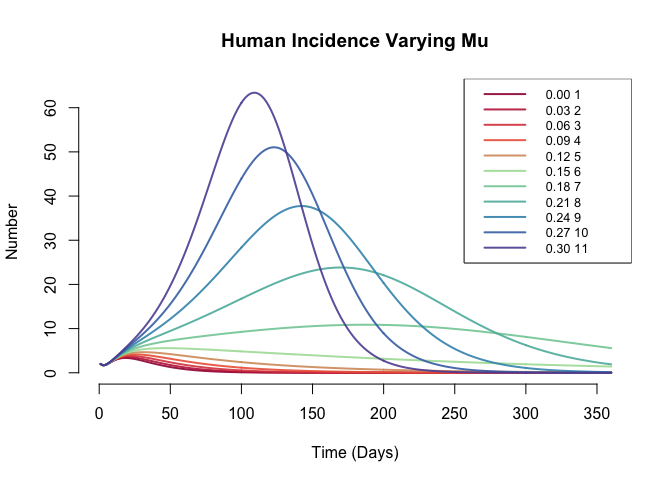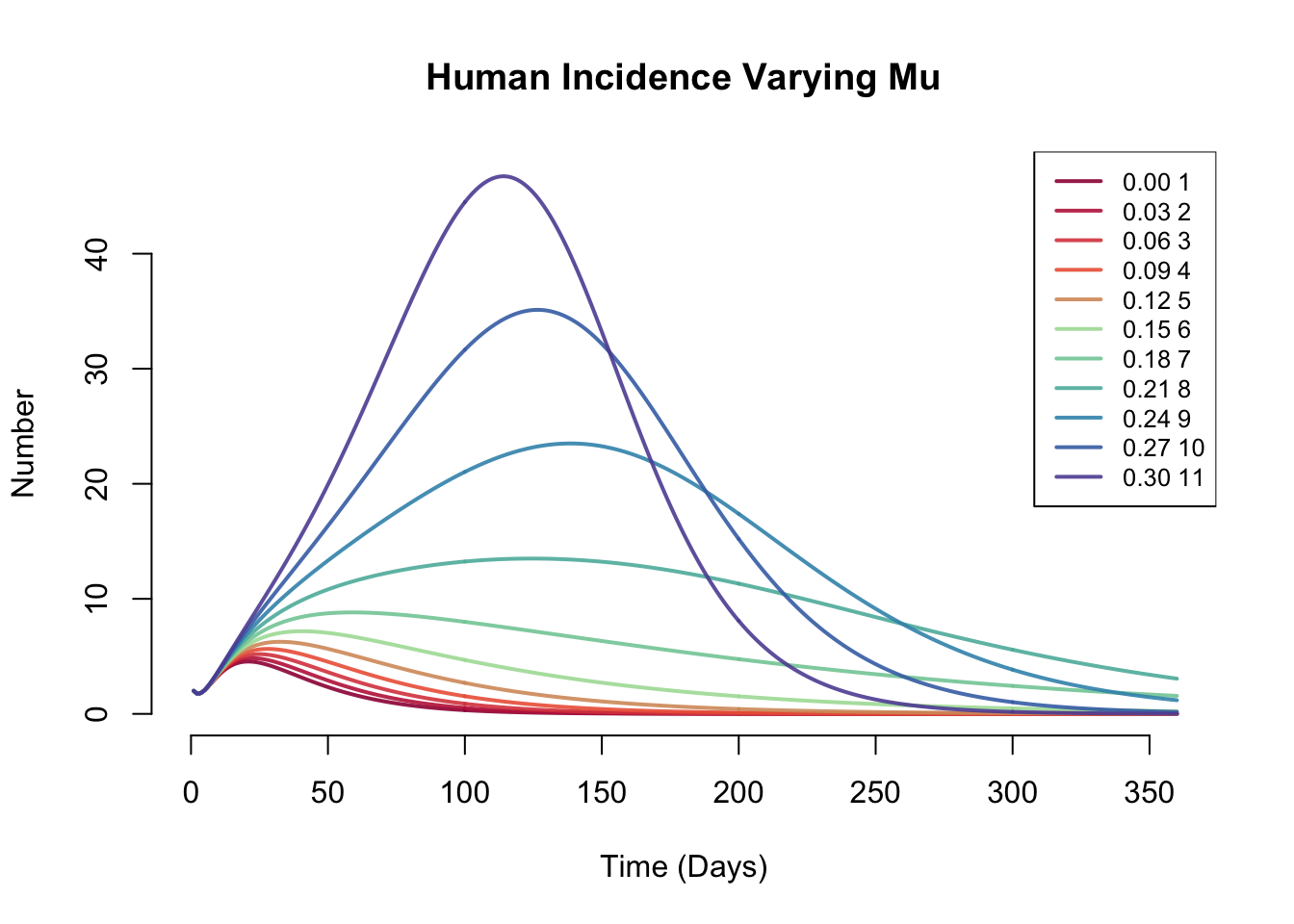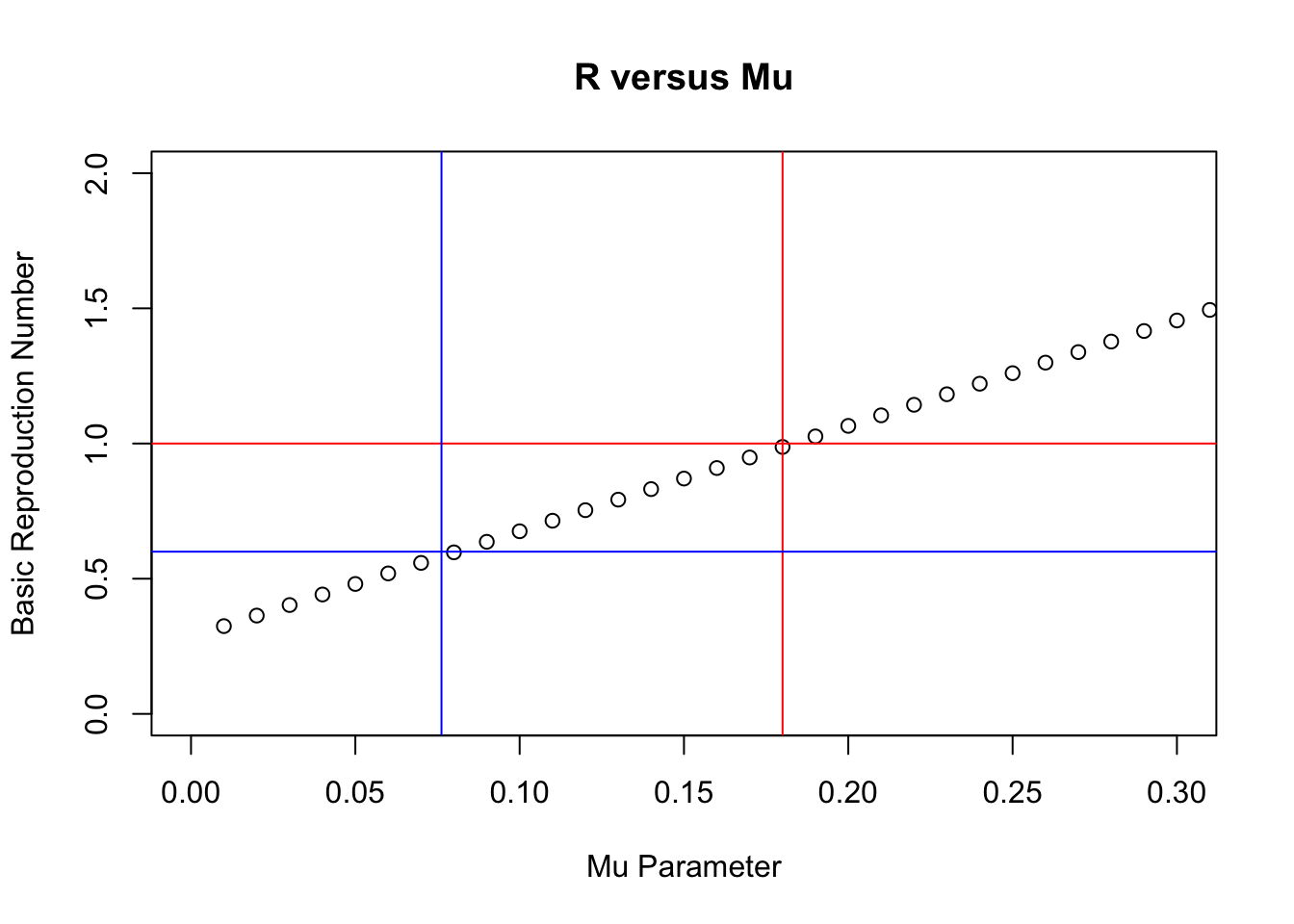# Nipah Virus ModelCurrently, outbreaks of Nipah virus disease among humans have been relatively small. To simulate Nipah virus pathogen evolution towards more effective human-to-human transmission capabilities, we assessed the impact on cumulative incidence and basic reproductive number (R0) of incremental increases in the proportion of the infectious humans that are highly infectious (Mu).

## Base model

``````library("EpiModel")
set.seed(12345)``````
``````Nipah <- function(t, t0, parms) { with(as.list(c(t0, parms)), {

# Derived parameters/states

num.b <- s_b.num + e_b.num + i_b.num + r_b.num
num.h <- s_h.num + e_h.num + i1_h.num + i2_h.num + r_h.num

lambda.bb <-  beta_BB * (i_b.num/num.b)
lambda.bh <-  beta_BH * (i_b.num/num.b)
lambda.h1 <- beta1_HH * (i1_h.num/num.h)
lambda.h2 <- beta2_HH * (i2_h.num/num.h)

# 1. Differential equations
dS_b <- -lambda.bb*s_b.num
dE_b <- lambda.bb*s_b.num - theta*e_b.num
dI_b <- theta*e_b.num - gamma*i_b.num
dR_b <- gamma*i_b.num

dS_h <- -lambda.h1*s_h.num - lambda.h2*s_h.num -lambda.bh*s_h.num
dE_h <-  lambda.h1*s_h.num + lambda.h2*s_h.num - sigma*e_h.num + lambda.bh*s_h.num
dI1_h <- mu*sigma*e_h.num - rho*i1_h.num
dI2_h <- (1-mu)*sigma*e_h.num - rho*i2_h.num
dR_h <- rho*(1-delta1)*i1_h.num + rho*(1-delta2)*i2_h.num
dD_h <- rho*delta1*i1_h.num + rho*delta2*i2_h.num

# 2. Outputs
list(c(dS_b, dE_b, dI_b, dR_b,
dS_h, dE_h, dI1_h, dI2_h, dR_h, dD_h,
si_h_BH.flow = lambda.bh*s_b.num,
si_h_HH.flow = lambda.h1*s_h.num + lambda.h2*s_h.num ))
})
}``````

### Parameters

``````param <- param.dcm(theta=1/6,
gamma=1/15,
sigma=1/9,
rho=1/6,
mu=seq(0.0, 0.3, 0.03), # Vary Mu from 0 to 0.3 in increments of 0.03
delta1=1,
delta2=0.71315524,
beta_BB=0.01436117,
beta_BH=0.11595493,
beta1_HH=0.69733082,
beta2_HH=0.04760139)

init <- init.dcm(s_b.num = 990, e_b.num=0, i_b.num = 10, r_b.num = 0,
s_h.num = 998, e_h.num=0, i1_h.num = 1, i2_h.num=1, r_h.num = 0, d_h.num = 0,
si_h_BH.flow=0, si_h_HH.flow=0)

control <- control.dcm(nsteps = 360, new.mod = Nipah)

sim <- dcm(param, init, control)``````

### Calculate Additional Values of Interest

``````sim <- mutate_epi(sim, h.total = s_h.num + e_h.num + i1_h.num + i2_h.num + r_h.num) # Total number of humans
sim <- mutate_epi(sim, i_h.total = i1_h.num + i2_h.num)                             # Total number of infected humans``````

## Figure 1: Human Incidence Varying Mu

Using an SEIR model with stratified compartments for highly infectious and less infectious humans and parameters calibrated to the 2018 Kerala outbreak, we ran 11 simulations varying the Mu parameter from 0.0 to 0.3 in increments of 0.03. This parameter governs the proportion of infectious individuals that are highly infectious (Mu) and less infectious (1 - Mu) with regards to their ability to spread Nipah virus to other humans. Plots of the incidence case series (epi curve) for 11 model runs with varying Mu were presented.## Figure 2: Cumulative Incidence by varying Mu parameter

Using the same 11 model simulations, we calculated the cumulative incidence attributable to human-to-human transmission of each outbreak scenario and plotted the cumulative incidence and Mu value for each simulation. Human-to-human attributable cumulative incidence was used (excluding incidence attributable to bat-human infections) in an effort to isolate the impact of human-to-human transmission by itself. For comparison to observational outbreak investigation, we also highlighted the cumulative incidence (23 cases) from the 2018 Kerala outbreak and Mu parameter calibrated to that outbreak from our model.

``````# Calculate the Cumulative Incidence for each run

# 1) Convert the model runs into data frames
df1 <- as.data.frame(sim, run = 1)
df2 <- as.data.frame(sim, run = 2)
df3 <- as.data.frame(sim, run = 3)
df4 <- as.data.frame(sim, run = 4)
df5 <- as.data.frame(sim, run = 5)
df6 <- as.data.frame(sim, run = 6)
df7 <- as.data.frame(sim, run = 7)
df8 <- as.data.frame(sim, run = 8)
df9 <- as.data.frame(sim, run = 9)
df10 <- as.data.frame(sim, run = 10)
df11 <- as.data.frame(sim, run = 11)

# 2) Calculate the cumulative incidence for each model run

# NOTE: Here we are only using Human-to-Human infections
# to calculate CI, because Bat-to-human infections are
# unaffected by varying Mu, and we are more interested
# in the dynamics of infections attributable to
# human-to-human transmission here.

ci1 <- sum(df1\$si_h_HH.flow)
ci2 <- sum(df2\$si_h_HH.flow)
ci3 <- sum(df3\$si_h_HH.flow)
ci4 <- sum(df4\$si_h_HH.flow)
ci5 <- sum(df5\$si_h_HH.flow)
ci6 <- sum(df6\$si_h_HH.flow)
ci7 <- sum(df7\$si_h_HH.flow)
ci8 <- sum(df8\$si_h_HH.flow)
ci9 <- sum(df9\$si_h_HH.flow)
ci10 <- sum(df10\$si_h_HH.flow)
ci11 <- sum(df11\$si_h_HH.flow)

# 3) Create data frame with cumulative incidence and varying Mu
mu <- seq(0.0, 0.3, 0.03)
ci <- rbind(ci1, ci2, ci3, ci4, ci5, ci6, ci7, ci8, ci9, ci10, ci11)
mu.by.ci <- as.data.frame(cbind(mu, ci))

plot(x=mu,
y=ci,
main = "Cumulative Incidence versus Mu",
xlab = "Mu",
ylab = "Cumulative Incidence")
abline(v=0.07621085) # Vertical line represents the calibrated Mu parameter for the 2018 Kerala Outbreak
abline(h=23)         # Horrizontal line represents the cumulative incidence for the 2018 Kerala Outbreak``````## Calculate R0

Finally, using the same 11 model simulations, we estimated the theoretical R0 for each outbreak scenario and presented a plot of R0 vs Mu. We highlighted the Mu parameter value at which R0 crosses the critical value (R0 = 1), and also the estimated R0 and Mu parameter values from the 2018 Kerala Outbreak.

R0 was calculated using the following base equation:

R0 = effective contact rate per unit time x duration of infection

Using a weighted average of the two Beta parameters:

R0 = ( Mu x (Beta1_HH) + (1 - Mu) x (Beta2_HH) ) x duration of infection

Though there are more precise ways to calculate R0 (ex. next generation matrices), we felt this was an adequate estimation method given that highly infectious and less infectious individuals mix randomly and only differ in the effectiveness of their contacts at producing transmission.

``````# 1) Use a weighted average of the calibrated beta parameters to calculate an R0 value at each value of Mu
R <- list()
for (i in 1:100) {
R[i] <- ((0.69733082*(i/100)) + (0.04760139*(1-(i/100))))*6
}

# 2) Make a sequence of values to be used for the x axis (Mu)
seq <- as.numeric(seq(0.01, 1, 0.01))``````
``````# Figure 3: R0 by varying Mu parameter

plot(x = seq,
y = R,
main = "R versus Mu",
ylab = "Basic Reproduction Number",
xlab = "Mu Parameter",
xlim = c(0,0.3),
ylim = c(0,2))
abline(h=1, col = "red")              # Represents the critical threshold for reproduction number (R=1)
abline(v=0.18, col = 'red')           # Represents the Mu parameter value at which R = 1
abline(h=0.60, col = "blue")          # Represents the R0 calculated in the literature for the 2018 Kerala Outbreak
abline(v=0.07621085, col = "blue")    # Represents the calibrated Mu parameter for the 2018 Kerala Outbreak``````# Discussion

Overall, increasing Mu substantially impacts the size and duration of human outbreaks of Nipah virus disease. At levels calibrated to the 2018 Kerala Outbreak (Mu = 0.076) our method predicts a similar cumulative incidence as was observed during that outbreak investigation (23 cases). However, with Mu increased to 0.3, cumulative incidence skyrockets to > 800 cases. These findings suggest that Nipah virus has not yet gained substantial human-to-human transmission capability.

Analysis of the cumulative incidence for outbreaks with increasing Mu also indicate that there is a threshold for Mu, above which human-to-human attributable cumulative incidence increases in a sigmoidal growth pattern. In our simulations, bat-human incidence was not impacted by variations in the Mu parameter, and the cumulative incidence due to bat-human transmissions was negligible (~20 additional cases in each simulation). Bat-to-human incidence was therefore excluded from the cumulative incidence analysis.

Finally, estimates of R0 from our simulations suggest that current Nipah outbreaks do not exhibit sustained transmission (R0 ≥ 1) and that they are unlikely to do so until Mu reaches or exceeds a threshold value (Mu ≥ 0.18). That said, Mu = 0.18 is not a particularly high threshold, and may be a plausible future value for Mu given that Nipah virus is evolving quickly, and has only recently developed the capability to infect humans and cause human-to-human transmission. However, seen another way, Mu = 0.18 represents a more than 2x increase in the proportion of humans that are highly infectious compared to currently observed values - an increase that would represent a drastic change in the way Nipah currently presents in populations. Further research on viral evolution would provide a clearer picture of the probability that Nipah virus might attain this capability in the future.##### Duncan Mahood
###### Epidemiology - Data Science - Web Development

Duncan Mahood is a public health consultant with Epidemiologic Research & Methods (ERM) and the Epidemiology Department at Rollins School of Public Health.